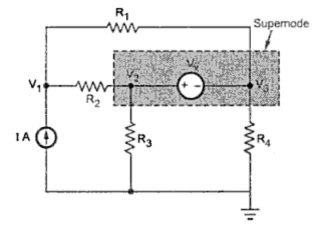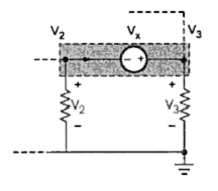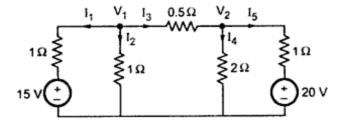### Node Analysis

This method is mainly based on Kirchhoff's Current Law (LCL). This method uses the analysis of the different nodes of the network. We have already defined a node. Every junction point in a network, where two or more branches meet is called a node. One of the nodes is assumed as reference node whose potential is assumed to be zero. It is also called zero potential node or datum node. At other nodes the different voltages are to be measured with respect to this reference node. The reference node should be given a number zero and then the equations are to be written for all other nodes by applying KCL. The advantage of this method lies in the fact that we get (n - 1) equations to solve if there are 'n' nodes. This reduces calculation work.
Consider the following network shown in the Fig. 1.
Let voltage at node 1 and node 2 be V1 and V2. Mark various branch currents as shown in the Fig.2. Now analyse ech node using KCL. independently.
Now applying KCL at node 1,
I1 - I3 - I4 = 0                                     ...........(1)
At node,       I2 + I4 - I5 = 0                                       ..........(2)
The currents in these equations can be expressed interms of node voltage as,
I1 - (V1 /R1)- (V1-V2/ R2) = 0               ...........(3)
and               I2 + (V1-V2/R2) - (V2/R3)= 0              ..............(4)
As I1 and I2 are known, we get two equations (3) and (4) with the two unknowns V1and V2. Solving these equations simultaneously, the node voltages V1and V2 can be determined. Once V1and V2 are known, current through any branche of the network can be determined. If there exists a voltage source in any of the branches as shown in the Fig.3 then that must be considered while writing the equation for the current through that branch.Fig. 2
Now V1 is at higher potential with respect to base, forcing current downwards. While polarity of Vx is such that it tries to force current upwards. So in such a case equation of current becomes,
I = (V1 - Vx)/R                                         ......... I leaving the node
If the direction of current I is assumed entering the node then it is assumed that Vx is more than V1 and hence equation for current I becomes,
I = (Vx - V1)/R                                        ........... I entering the node

1.1 Points to Remember for Nodal Analysis
1. While assuming branch currents, make sure that each unknown branch current is considered at least once.
2. Convert the voltage source present into their equivalent current sources for node analysis, wherever possible.
3. Follow the same sign conversion, currents entering at node are be considered positive, while currents leaving the node are to be considered as negative.
4. As far as possible, select the directions of various branch currents leaving the respective nodes.
1.2 Supernode
Consider a circuit shown in the Fig. 4. In this circuit, the nodes labelled V2 and V3 are connected directly through a voltage source, without any circuit element. The region surrounding a voltage source which connects the two nodes directly is called supernode.Fig. 4  Region of supernode
In such a case, the nodes in supernodes region can be analysed separately and the relation between such node voltages and a source voltage connecting them can be separately obtained. In the circuit shown in the Fig.4 we can write,
V2 = V3+ Vx
In addition to this equation, apply KCL  to all the nodes assuming different branch currents at the nodes. The current through voltage source, connecting supernodes must be expressed interms of node voltages, using these KCL. equations. Then the resulting equations and supernode equations are to be solved simultaneously to obtain the required unknown.
Now consider the loop including supernode as shown in the Fig. 5.Fig. 5  Loop including supernode
Applying KVL to the loop,
- Vx- V3 + V2 = 0
V2 = Vx + V3
Thus the relationship between supernode voltages can be obtained using KVL.
Such equation can be written by inspection also. For the Fig. 6 shown, the equation is,
V= V2 + Vx
This is because V is helping V2 to force current from node 2 to node 3.Fig. 6
1.3 Steps for the Node Analysis
Step 1 : Choose the nodes and node voltages to be obtained.
Step 2 : Choose the currents preferably leaving the node at each branch connected to each node.
Step 3 : Apply KCL at each node with proper sign convection.
Step 4 : If there are supernodes, obtain the equations directly interms of voltages which are directly connected through voltage source.
Step 5 : Obtain the equation for the each branch current interms of node voltages and substitute in the equations obtained in step 3.
Step 6 : Solve all the equations obtained in step 4 and step 5 simultaneously to obtain the required node voltages.
Key Point : If there are many number of branches in parallel in a network then node method is advantageous for the network analysis.

Example 1 : Find the current through each resistor of the circuit shown in the Fig. 7, using node analysisFig. 7
Solution ; The various node voltages and currents are shown in the Fig. 7(a)Fig. 7(a)
At node 1,            -I1 -I2 -I3 = 0
-(V1-15 /1) - (V1/1) + (V1-V2 /0.5)=  0
-V1 + 15 - V1 - 2V1 + 2V2= 0
4V1 - 2V2 = 15                                      .........(1)
At node 2,             I3 - I4 - I5 = 0
(V1-V/0.5) - (V2/2) - (V2-20 /1) = 0
2V1 - 2V2 - 0.5V2 - V2 + 20 = 0
2V1 - 3.5 V2 = -20                                .............(2)
Multiplying equations (2) by 2 and subtracting from equation (1) we get,
5V2= 55
V2 = 11 V
and                          V1 = 9.25 V
Hence the various currents are,
I1= (V1- 5)/1 = 9.25 - 15 = -5.75 A i.e. 5.75 A
I2 = V1/1 = 9.25 A
I3 = (V1-V2)/0.5 = -3.5 A i.e. 3.5 A
I4 = V2/2 = 5.5 A
I5 = (V2 - 20)/1 = (11-20)/1 = -9 A i.e. 9 A
Example 2 : Determine the node voltages for the network shown by nodal analysis Fig.8.Fig. 8
Solution : The various node voltages are shown in the Fig. 8(a).Fig. 8(a)
The various branch currents are shown. Applying KCL at various nodes.
Node 1 :                9 - I1 - I2 - I3 = 0                    .........(1)
Node 2 :                      I3 - I4 + 4 = 0                      .........(2)
Node 3 :                      I- 4 - I5 = 0                     ..........(3)
Key Point : Nodes V1 and V2 from supernode region and nodes V1 and V2 from super node region.
Super node :             V1 - 10 = V3   i.e. V1 - V3 = 10           .........(4)
Super node :             V1 + 6 =       i.e. V1 - V2 = -6               ........(5)
From equation (2),     I3 = I4 - 4 and from equation (3), I2 = I4 + 4
Using in equation (1),  9- I1 - I5 - 4 - I4 + 4 = 0
i.e.                              I1 + I4 + I5 = 9           .........(6)
But                              I1 = V1/4,    I4 = V2/10,         I5 = V3/5
(V1/4) + (V2/10)+ (V3/5) = 9
0.25 V1 + 0.1 V2 + 0.2 V3 = 9               ..........(7)
Solving equations (4), (5) and (7) simultaneously,
V1= 18.909 V,     V2= 24.909 V,       V3= 8.909 V